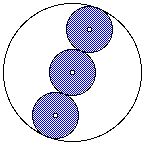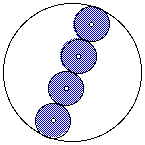# Tangent Along the DiameterAlong the diameter of a circle you can construct circles with equal radii that are tangent to each other. The outermost circles in the string of circles will be tangent to the large circle. (Tangent means that the circles touch each other but do not cross over each other, nor do they leave any gaps.)

How does the combined area of all of the shaded circles relate to the area of the entire circle?Extension:
If you were to walk along the entire circumferences of all the small circles, would you walk farther or less far than if you walked around the circumference of the largest circle? How much more or how much less would you walk?